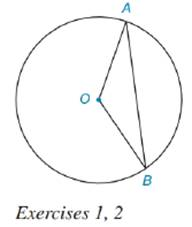Chapter 8.5, Problem 1E### Elementary Geometry for College St...

6th Edition
Daniel C. Alexander + 1 other
ISBN: 9781285195698

#### Solutions

Chapter
Section### Elementary Geometry for College St...

6th Edition
Daniel C. Alexander + 1 other
ISBN: 9781285195698
Textbook Problem
7 views

# Given ⊙ O with radii O A - and O B - and chord A B - .a) What type of figure (sector or segment) is bounded by O A - , O B - and A B ^ ?b) If O A = 7 cm and l A B ^ = 11 cm, find the perimeter of the figure in (a).To determine

a. To find:

The type of figure bounded by OA-, OB- and AB^

Explanation

Sector is a pie shaped part of the circle enclosed by two radii and their intercepted arc.

In the given diagram, OA- and OB- are the radii of circle with centre O. AB^ is the arc intercepted by the radii. Thus, the figure bounded by radii OA-, <

To determine

b. To find:

The perimeter of figure in (a).

### Still sussing out bartleby?

Check out a sample textbook solution.

See a sample solution

#### The Solution to Your Study Problems

Bartleby provides explanations to thousands of textbook problems written by our experts, many with advanced degrees!

Get Started